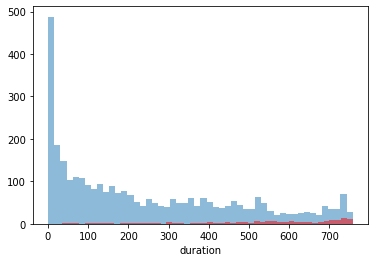Full replication
INFO:tensorflow:Enabling eager execution
INFO:tensorflow:Enabling v2 tensorshape
INFO:tensorflow:Enabling resource variables
INFO:tensorflow:Enabling tensor equality
INFO:tensorflow:Enabling control flow v2

This notebook reproduces every table in LaPlante et al's 2009 paper on poker player behaviour. To get started, download the raw data (Sitting at the Virtual Poker Table) from the link below from the transparency project's website;

File names: DS4_Raw2_Aggregate_txt.txt and DS4_Analytic_txt.txt

With the data downloaded, the first step is to import gamba, run the cell below to get started;Data description above mentions 'Eligibility for poker analysis' as a variable in the daily aggregate data which is not present. This is not a fatal discrepency, and can be retrospectively added using player's existence in the analytical data set.Both the Cohen's d and K-S test results computed below differ from the original paper, this is likely due to differences in the ways these tests are computed or an undocumented data transformation (see third flag).Paper contains undocumented results - df, t, df (again), p, and p(2-tailed). These are only referenced in the sentence 'Tests for differences between group means included testing the assumption of equal variances and, if necessary, adjusting for unequal variances.`

import gamba as gb

With gamba ready, we need to load in both the analytic and raw data sets from the link above - we need both to make sure that the analytic data set is indeed a subset of the raw data set;

Next, taking only the analytic data forward (for now), we can prepare the three subsets described in the paper (full set, most involved, not most involved);

useful_columns = ['UserID','Duration','Total_sessions','Sessions_Per_Day','Euros_Per_Session','Total_wagered','Net_Loss','Percent_Lost']
all_study_data = analytic_data[useful_columns] # get only columns that are used in the analysis, then rename them to be compatable with gamba
all_study_data.columns = ['player_id','duration','total_sessions','sessions_per_day','euros_per_session','total_wagered','net_loss','percent_loss']

# now do the same for the 5-95 split of players performed in the study (using the 'Most_Involved_Group' column)
most_involved = analytic_data[analytic_data['Most_Involved_Group'] == 1]
most_involved = most_involved[useful_columns]
most_involved.columns = ['player_id','duration','total_sessions','sessions_per_day','euros_per_session','total_wagered','net_loss','percent_loss']

not_most_involved = analytic_data[analytic_data['Most_Involved_Group'] == 0]
not_most_involved = not_most_involved[useful_columns]
not_most_involved.columns = ['player_id','duration','total_sessions','sessions_per_day','euros_per_session','total_wagered','net_loss','percent_loss']

print('all players:', len(all_study_data))
print('most involved:', len(most_involved))
print('others 95 percent:', len(not_most_involved))
all players: 3445
most involved: 173
others 95 percent: 3272

These numbers are identical to those found in the paper, so at this stage we can be reasonably sure that the data extracted above is the same as that used in the original analysis. Using gamba we can then reproduce each of the tables with the descriptive_table function, plus the cohens_d and ks_test functions...

descriptive_df = gb.statistics.descriptive_table(all_study_data)
ks_table = gb.statistics.ks_test(all_study_data)
combined = gb.data.concat([descriptive_df, ks_table], axis=1)
display(combined)

majority_table = gb.statistics.descriptive_table(not_most_involved)
minority_table = gb.statistics.descriptive_table(most_involved)
display(majority_table, minority_table)

most_involved['most_involved'] = 1
not_most_involved['most_involved'] = 0
all_labelled_data = gb.data.concat([most_involved, not_most_involved])
cohens = gb.statistics.cohens_d(all_labelled_data, 'most_involved')
most_involved.drop(['most_involved'], axis=1, inplace=True)
display(cohens)

spearmans = gb.statistics.spearmans_r(all_study_data)
second_spearmans = gb.statistics.spearmans_r(most_involved)
display(spearmans)
display(second_spearmans)
mean std median K-S Score p
duration 263.297242 231.765320 196.000000 0.962656 0.0
total_sessions 197.189550 376.112854 60.000000 0.999968 0.0
sessions_per_day 1.141501 1.639888 0.560976 0.502543 0.0
euros_per_session 34.822931 186.660876 13.150878 0.965538 0.0
total_wagered 8684.051892 83583.345565 807.989800 0.999706 0.0
net_loss 587.460887 3018.728832 110.659371 0.873454 0.0
percent_loss 0.239377 0.294077 0.204473 0.424153 0.0
mean std median
duration 249.192848 225.160554 181.000000
total_sessions 151.582213 256.414753 52.000000
sessions_per_day 1.084689 1.607911 0.506123
euros_per_session 24.473024 45.616327 12.323661
total_wagered 2736.739945 4784.441999 703.815150
net_loss 465.789054 1210.518983 105.846000
percent_loss 0.248375 0.297668 0.214518
mean std median
duration 530.057803 189.896505 565.000000
total_sessions 1059.774566 891.173874 818.000000
sessions_per_day 2.216007 1.859378 1.616105
euros_per_session 230.573760 785.814580 88.660873
total_wagered 121167.315999 355037.671438 55012.673500
net_loss 2888.676138 12206.189893 1941.010535
percent_loss 0.069203 0.126085 0.033964
Cohen's d
duration -1.256492
total_sessions -2.841726
sessions_per_day -0.697742
euros_per_session -1.137564
total_wagered -1.489859
net_loss -0.815126
percent_loss 0.614648
duration total_sessions sessions_per_day euros_per_session total_wagered net_loss percent_loss
duration -
total_sessions 0.62** -
sessions_per_day -0.38** 0.37** -
euros_per_session 0.12** 0.09** -0.03 -
total_wagered 0.56** 0.85** 0.29** 0.57** -
net_loss 0.31** 0.44** 0.1** 0.42** 0.59** -
percent_loss -0.23** -0.41** -0.18** -0.07** -0.36** 0.36** -
duration total_sessions sessions_per_day euros_per_session total_wagered net_loss percent_loss
duration -
total_sessions 0.34** -
sessions_per_day -0.22** 0.76** -
euros_per_session -0.21** -0.74** -0.58** -
total_wagered 0.09 0.07 -0.01 0.55** -
net_loss -0 -0 0.03 -0.13 -0.17* -
percent_loss -0.03 0 0.08 -0.18* -0.28** 0.95** -

That's it! The tables found in the paper can be recreated from those above - minus a df, t, p, and p(2-tailed) column which are not described in the text.

This short population-level descriptive analysis lays the foundation for work in other areas/with other providers. For more examples of reproducability using gamba, visit the github repo or click through the replications tab on the left.

measure = 'duration'
control = not_most_involved[measure].values
experimental = most_involved[measure].values

import matplotlib.pyplot as plt
plt.figure()
plt.hist(control, alpha=0.5, bins=50)
plt.hist(experimental, color='red', alpha=0.5, bins=50)
plt.xlabel(measure)
plt.show()